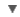POS
WORDNET DICTIONARY
CIDE DICTIONARY
OXFORD DICTIONARY
Link, Gadget and Share#erg

:
Noun

## CIDE DICTIONARY

ergn. [Gr. work.].
The unit of work or energy in the C. G. S. system, being the amount of work done by a dyne working through a distance of one centimeter; the amount of energy expended in moving a body one centimeter against a force of one dyne (981 dynes exert the same force as a one gram mass in the earth's gravitational field). One foot pound is equal to 13,560,000 ergs. The absolute Joule is equivalent to 107 ergs, which are equivalent to 0.2389 gram-calories at 15mechanical equivalent of heat under equivalent.  [1913 Webster]

## OXFORD DICTIONARY

erg, n. Physics a unit of work or energy, equal to the work done by a force of one dyne when its point of application moves one centimetre in the direction of action of the force.

erg, n. (pl. ergs or areg) an area of shifting sand-dunes in the Sahara.

Etymology
F f. Arab. ' irj
See related words and definitions of word "erg" in Indonesiancopyright © 2012 Yayasan Lembaga SABDA (YLSA) | To report a problem/suggestion